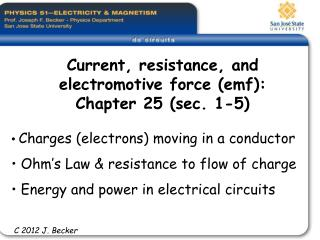DownloadDownload PresentationCurrent, resistance, and electromotive force (emf): Chapter 25 (sec. 1-5)

# Current, resistance, and electromotive force (emf): Chapter 25 (sec. 1-5)

Télécharger la présentation## Current, resistance, and electromotive force (emf): Chapter 25 (sec. 1-5)

- - - - - - - - - - - - - - - - - - - - - - - - - - - E N D - - - - - - - - - - - - - - - - - - - - - - - - - - -
##### Presentation Transcript

1. Current, resistance, and electromotive force (emf): Chapter 25 (sec. 1-5) • Charges (electrons) moving in a conductor • Ohm’s Law & resistance to flow of charge • Energy and power in electrical circuits C 2012 J. Becker

2. Learning Goals - we will learn: • The meaning of electric current, and how electric charges move in a conductor.• What is meant by the resistivity of a substance.• How to calculate the resistance of a conductor from its dimensions and its resistivity.• How an electromotive force (emf) makes it possible for current to flow in a circuit.• How to do calculations involving energy and power in circuits.

3. ANALOGY OF A CHARGE MOVINGIN A CONDUCTOR 12 Volts 0 Volts

4. CONDUCTOR WITH CONVENTIONAL CURRENT MOVING FROM HIGH ELECTRICAL POTENTIAL (VOLTS) TO LOW POTENTIAL

5. “CONVENTIONAL” CHARGESDRIFTING IN A CONDUCTOR HIGHER POTENTIAL LOWER POTENTIAL LAMP

6. Which Box (A, B, or C) has the most resistance to the flow of electric charge (current)? Each lamp has the same amount of resistance to the flow of charge. Current is the flow of charge past a point in the circuit per unit time interval. C 1998 McDermott, et al., Prentice Hall

7. Which network has the most resistance to the flow of charge? Rank the networks according to decreasing resistance. C 1998 McDermott, et al., Prentice Hall

8. Rank the brightness of the bulbs (bright to dim). 2. A wire is added as shown below. • Does the brightness of bulb C increase, decrease, or remain the same? • Does the brightness of bulb A increase, decrease, or remain the same? • Does the current through the battery increase, decrease, or remain the same? C 1998 McDermott, et al., Prentice Hall

9. Resistance (R) is proportional to resistivity (r): R = r L / AThe resistivity (r) depends on temperature and the physical properties of the material, so it has a different value for each material. Temperature dependence of resistance (and resistivity) is generally linear over limited temperature ranges and is characterized by the temperature coefficient of resistivity (a): R(T) = R0 [ 1 + a (T-T0)] r(T) = r0 [ 1 + a (T-T0)] where R0 and T0 are the resistance and temperature at a standard temperature, usually room temperature or 20o C. (Measured in Lab #5)

10. Current – voltage relations • a resistor obeys Ohm’s Law: I = V/R with constant slope = 1/R (or DV = I R) b) A vacuum tube diode c) A semiconductor diode

11. Electric potential (DV) rises and drops in a circuit (from previous slide)

12. CIRCUIT ENERGY and POWER P = Vab I = I2 R = Vab2/ Re I = rate of conversion of non-electrical (chemical) energy to electrical energy within the sourceI2 r = rate of electrical energy dissipation in the internal resistance of the source (battery)e I - I2 r = the rate at which the source delivers electrical energy to the load (headlight) R

13. You can view a 4-minute youtube video of a smoke detector and how it works, from classmate Marjo Mallari http://www.youtube.com/watch?v=oFUUQcpGR3k